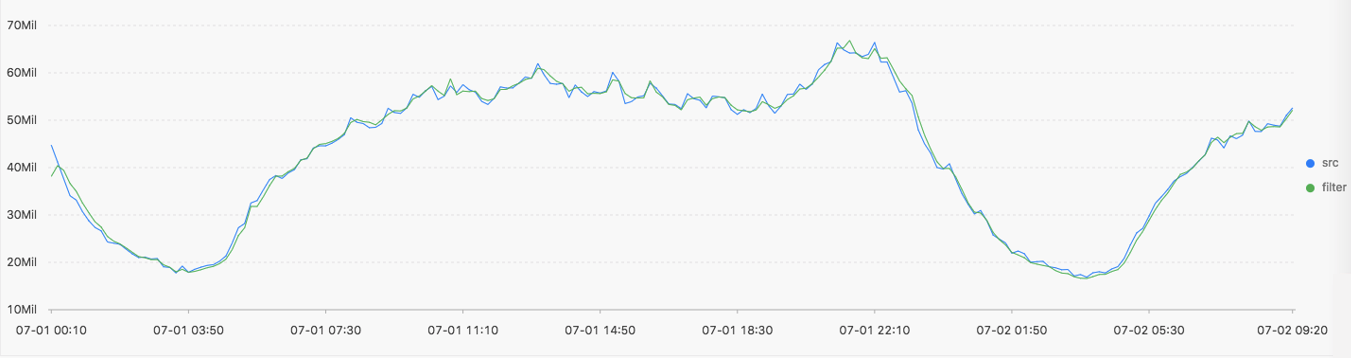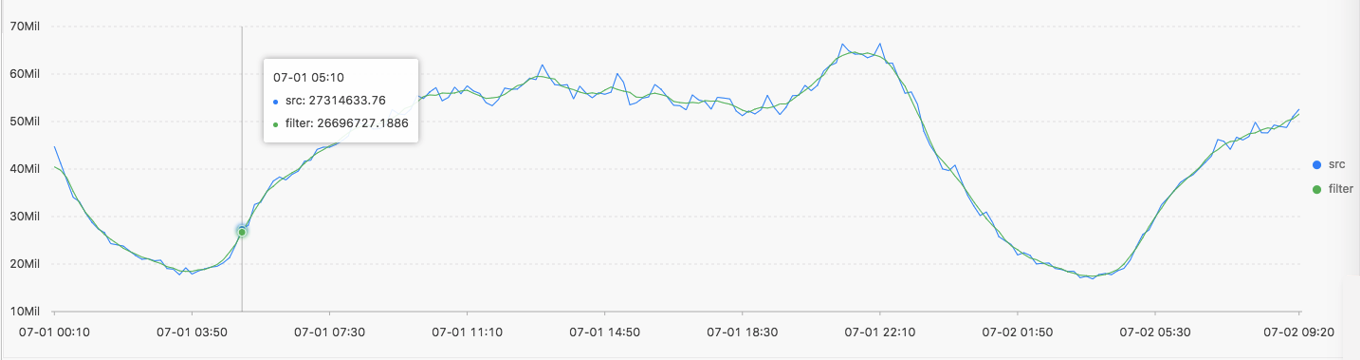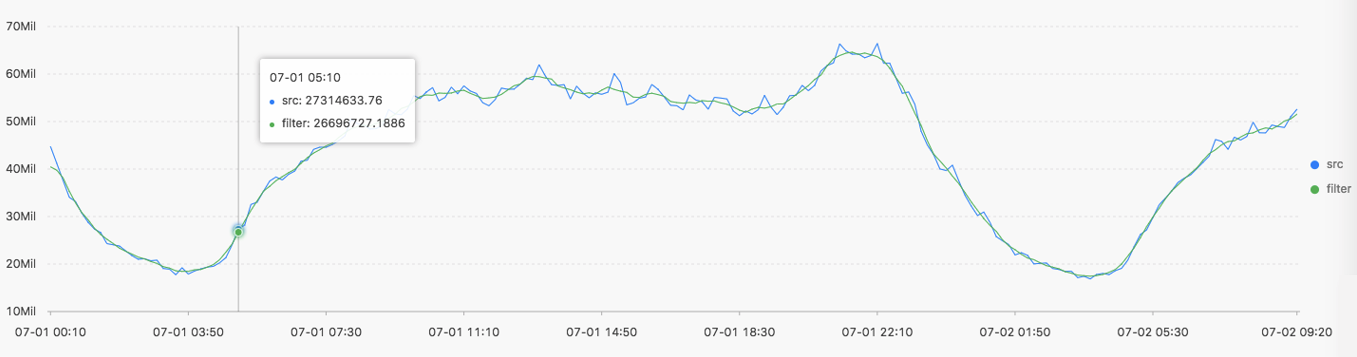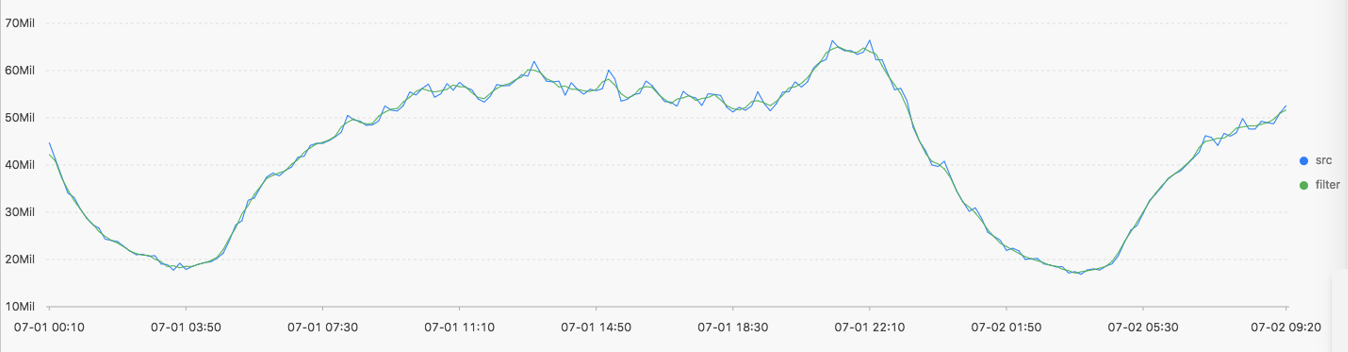This topic describes the smooth functions that you can use to smooth and filter specified time series curves. Filtering is the first step to discover the shape of time series curves.

## Function list

Function Description
`ts_smooth_simple` Uses the Holt-Winters forecasting algorithm to filter time series data. This function is the default smooth function.
`ts_smooth_fir` Filters time series data by using a finite impulse response (FIR) filter.
`ts_smooth_iir` Filter time series data using an infinite impulse response (IIR) filter.

## ts_smooth_simple

• Function format:
``select ts_smooth_simple(x, y)``
• The following table lists the parameters of the function format.
Parameter Description Value
x The time sequence. Points in time are sorted in ascending order along the horizontal axis. Each point in time is a Unix timestamp. Unit: seconds.
y The sequence of numeric data corresponding to each specified point in time. N/A.
• Examples
• The query statement is as follows:
``* | select ts_smooth_simple(stamp, value) from ( select __time__ - __time__ % 120 as stamp, avg(v) as value from log GROUP BY stamp order by stamp )``
• Output result• The following table lists the display items.
Display item Description
Horizontal axis unixtime Each point in time is a Unix timestamp. Unit: seconds.
Vertical axis src The raw data.
filter The data generated after the filtering operation is performed.

## ts_smooth_fir

• Function format:
• If you cannot determine filter parameters, use the built-in window parameters in the following statement:
``select ts_smooth_fir(x, y,winType,winSize)``
• If you can determine filter parameters, you can set the parameters as needed in the following statement:
``select ts_smooth_fir(x, y,array[])``
• The following table lists the parameters of the function format.
Parameter Description Value
x The time sequence. Points in time are sorted in ascending order along the horizontal axis. Each point in time is a Unix timestamp. Unit: seconds.
y The sequence of numeric data corresponding to each specified point in time. N/A.
winType The type of window for filtering. Valid values:
• rectangle: a rectangular window
• hanning: a Hanning window
• hamming: a Hamming window
• blackman: a Blackman window
Note We recommend that you set this parameter to rectangle for better display.
winSize The length of the filter window. The value is of the long data type. Valid values: 2, 3, 4, 5, 6, 7, 8, 9, 10, 11, 12, 13, 14, and 15.
array[] Used to calculate coefficients for the FIR filter. The value is an array where the sum of elements is 1. Example: array[0.2, 0.4, 0.3, 0.1].
• Example 1
• The query statement is as follows:
``* | select ts_smooth_fir(stamp, value, 'rectangle', 4) from ( select __time__ - __time__ % 120 as stamp, avg(v) as value from log GROUP BY stamp order by stamp ) ``
• Output result• Example 2
• The query statement is as follows:
``* | select ts_smooth_fir(stamp, value, array[0.2, 0.4, 0.3, 0.1]) from ( select __time__ - __time__ % 120 as stamp, avg(v) as value from log GROUP BY stamp order by stamp ) ``
• Output result• The following table lists the display items.
Display item Description
Horizontal axis unixtime Each point in time is a Unix timestamp. Unit: seconds.
Vertical axis src The raw data.
filter The data generated after the filtering operation is performed.

## ts_smooth_iir

• Function format:
``select ts_smooth_iir(x, y, array[], array[] ) ``
• The following table lists the parameters of the function format.
Parameter Description Value
x The time sequence. Points in time are sorted in ascending order along the horizontal axis. Each point in time is a Unix timestamp. Unit: seconds.
y The sequence of numeric data corresponding to each specified point in time. N/A.
array[] Used to calculate the filter coefficients related to x i for the IIR filter. The value is an array where the sum of elements is 1. Valid lengths of elements: 2, 3, 4, 5, 6, 7, 8, 9, 10, 11, 12, 13, 14, and 15. Example: array[0.2, 0.4, 0.3, 0.1].
array[] The type of the filter that specifies the algorithm to compute the filter coefficients related to y i-1 for the IIR filter. The value is an array where the sum of elements is 1. Valid lengths of elements: 2, 3, 4, 5, 6, 7, 8, 9, 10, 11, 12, 13, 14, and 15. Example: array[0.2, 0.4, 0.3, 0.1].
• Examples
• The query statement is as follows:
``* | select ts_smooth_iir(stamp, value, array[0.2, 0.4, 0.3, 0.1], array[0.4, 0.3, 0.3]) from ( select __time__ - __time__ % 120 as stamp, avg(v) as value from log GROUP BY stamp order by stamp )``
• Output result• The following table lists the display items.
Display item Description
Horizontal axis unixtime Each point in time is a Unix timestamp. Unit: seconds.
Vertical axis src The raw data.
filter The data generated after the filtering operation is performed.